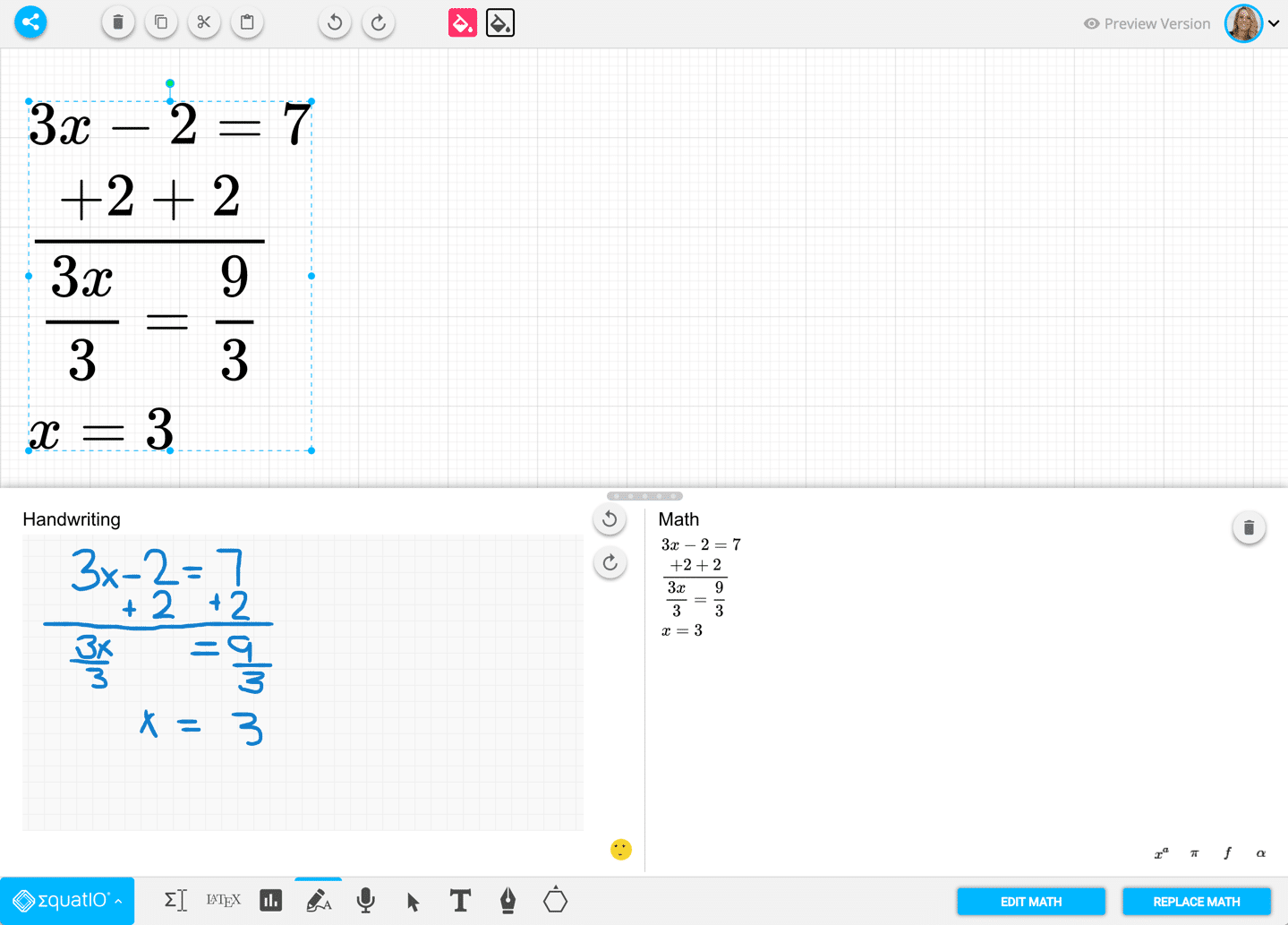*Update: EquatIO is now FREE for teachers!

# EquatIO, by Texthelp, is now available in the Chrome Webstore.

EquatIO is a Chrome extension that will allow you and your students to create math equations in Google Apps…. and other places. Today’s release only works in Google Docs and Google Forms but Google Slides and other web content sites will soon be able to utilize EquatIO.

## Math Type

As a math teacher if what you’re most concerned about is making digital symbols, you are missing the point.

Using technology in math is not about taking the same 100+ year old math problems and putting them online. Being able to insert math equations into Google Docs/Forms (and soon Slides) is definitely exciting, but is it more engaging for students? Same math digitally is the same learning and the same engagement.

How do we engage students in math? Have them WANT to talk about it? Understand math, increase fluency, increase critical thinking?

Most of the math problems in the textbook are DOK 1; low critical thinking. They are mainly about following procedural steps. While students can use EquatIO to show their steps, this is a skill that has literally been made obsolete by technology. Have students use EquatIO to show their THINKING, UNDERSTANDING, and CONNECTIONS.

## Handwriting Recognition

EquatIO allows for handwriting input to convert to math expressions.

## Voice Recognition

Ask the universe and the Quadratic formula appears magically! EquatIO does that.

## Prediction

EquatIO predicts what you want making math insertion significantly faster and easier.

## Geometry Symbols without a Pallette

Type the geometry symbol you want “angle” and it is converted into the symbol.

EquatIO works in Google Slides! I am a big fan of using Google Slides for math because of the collaboration, the open canvas space to provide multi media, the ability to have feedback conversations and peer feedback, and more!

## Mathspace

Texthelp has upgraded EquatIO with a product called EquatIO Mathspace. This is an open canvas to insert math as well as shapes and images to allow students to model mathematics. Access it through equat.io## Subscribe to the Alice Keeler Newsletter